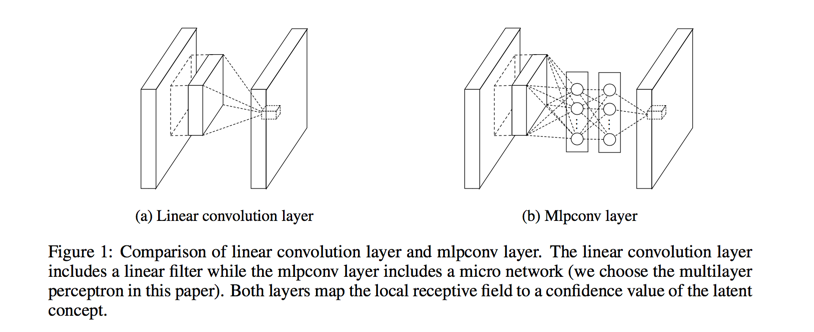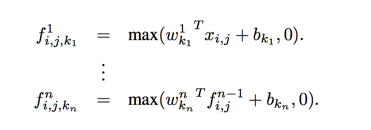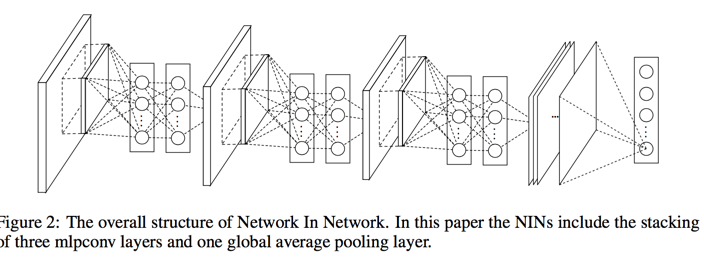Network in Network

Posted by GwanSiu on May 20, 2017

1.What’s the network in network? (An brief introduction)

• Replace convolutional operator with a “micro-network”. In this article, the convolutional operator is considered as general operator and utilize multi-layer percetron as “micro-network”.

• Replace fully-connected layers with global average pooling layers. The purpose of fully-connected layers is to map a feature map to a vector for classification, but the fully-connected layers is prone to overfit. In tradictional arichitecture of CNN, the fully-connected layers is usually followed by the dropout layers to prevent from overfitting.

2.The arichitecture of the network in network3.Why can the convolutional operator be replaced by a “micro-network”?

3.1 The conventional neural network

The classical neural network is consist of alternatively the stack of convolutional filter and spatial pooling layers. The feature maps are generated by the conlutional filters which is followed by the activation functions, the feature map can be calculated as follows(linear rectifier function):

Here $(i,j)$ is the pixel index in the feature map, $x_{i,j}$ stands for the input patch centered at location $(i, j)$, and $k$ is used to index the channels of the feature map.

The convolutional filter is considered as a general linear model for underlying local patch. Those convolutional filters can extracture invariant features from low level pixel. Indicidual linear filters can learn different vaiations of a same concept. The linear filters in the next layer will consider all combinations of variations from the previous layer. At this point, we should note GLM can achieve a good extent of abstraction when the samples of the latent concepts are linearly separable, i.e. the variants of the concepts all live on one side of the separation plane defined by the GLM. The assuption behind the convolutional operator is that the latent concepts are linearly separable. However, the conventional linear filters impose a heavyburden on the neural network.

3.2 why is the micro-network used?

what we hope? Given no priors about the distributions of the latent concepts, it is desirable to use a universal func- tion approximator for feature extraction of the local patches, as it is capable of approximating more abstract representations of the latent concepts.

The “micro-network” is considered as a more general linear model which is capable of approxmating any convex functions. (which is more looser than the conventional neural network). Why does MLP used? 1. MLP is compatible wirh the strure of the convolutional neural work, which is trained using back-propagation. 2. MLP can be a deep model itself, which is consistent with the spirit of feature re-use.Here $n$ is the number of layers in the multilayer perceptron. Rectified linear unit is used as the activation function in the multilayer perceptron.

From cross channel(cross featiure map) pooling point of view, this equation is equivalent to cascade cross channel parametric pooling on a normal convolutional layer. For example, 1x1 convolutional kernel is a linear combinations of the cross channels.

3.3 what’s the global average pooling operator

Global average pooling operator is to generate one feature map for each corresponding category of the classification task in the last mlpconv layer. This designed structure has no parameters to optimize thus overfitting is avoided at this layer.

3.4 the overall structure of network in network